# Atomic Structure IPage 1

#### WATCH ALL SLIDES

Slide 1## Atomic Structure

Slide 2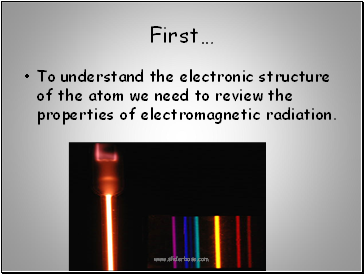First…

To understand the electronic structure of the atom we need to review the properties of electromagnetic radiation.

Slide 3Figure 7.1

Frequency and Wavelength

c = l n

l = wavelength

n = frequency

C = speed of light

The Wave Nature

of Light

Slide 4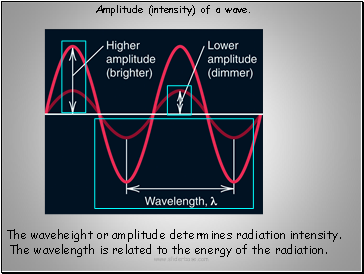The waveheight or amplitude determines radiation intensity.

The wavelength is related to the energy of the radiation.

Slide 5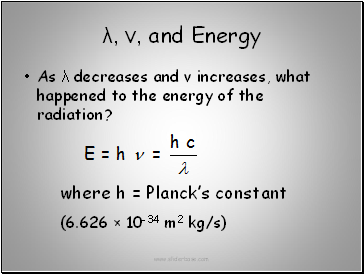λ, ν, and Energy

As λ decreases and ν increases, what happened to the energy of the radiation?

where h = Planck’s constant

(6.626 × 10-34 m2 kg/s)

Slide 6Regions of the electromagnetic spectrum.

The infinite number of wavelengths of

into groups as shown below.

Slide 7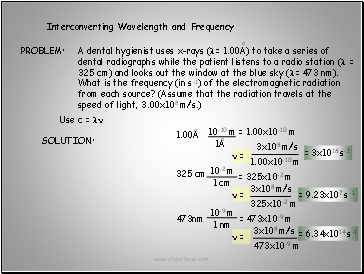SOLUTION:

Interconverting Wavelength and Frequency

Use c = ln

= 1.00x10-10 m

= 325x10-2 m

= 473x10-9 m

n =

3x108 m/s

1.00x10-10 m

= 3x1018 s-1

n =

n =

3x108 m/s

325x10-2 m

= 9.23x107 s-1

= 6.34x1014 s-1

Slide 8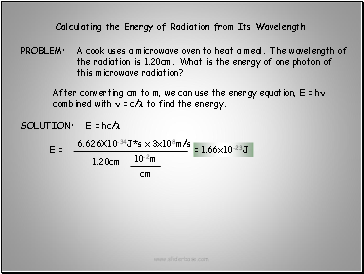SOLUTION:

Calculating the Energy of Radiation from Its Wavelength

After converting cm to m, we can use the energy equation, E = hn combined with n = c/l to find the energy.

E = hc/l

E =

6.626X10-34J*s

3x108m/s

1.20cm

x

= 1.66x10-23J

Slide 9## Particle or Wave?

Different behaviors of waves and particles.

Slide 10The diffraction pattern caused by light

Slide 11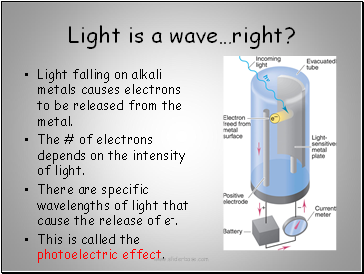## Light is a wave…right?

Light falling on alkali metals causes electrons to be released from the metal.

The # of electrons depends on the intensity of light.

There are specific wavelengths of light that cause the release of e-.

This is called the photoelectric effect.

Slide 12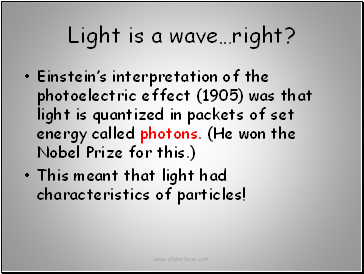Go to page:
1  2  3